TEL : +81 48 254 1744

"Easy" Gear Selection Screen

- Choose a gear by pitch, # of teeth, or bore size.
- 2D/3D Drawings Available
- PDF Catalog Available
- Strength Calculation Available

Go to Gear Selection Screen

CLOSE

# Gear Trains

Gears cannot work singularly to transmit power. At least two or more gears must be meshed to work. This section introduces a simple gear train “Single-Stage Gear Train” and its use in pairs for a “Two-Stage Gear Train”.

## 2.1 Single-Stage Gear Train

A pair of meshed gears is the basic form of a single-stage gear train. Figure 2.1 shows forms of the single-stage gear train.

In a single-stage gear train, which consists of z1 and z2 numbers of teeth on the driver and driven gears, and their respective rotations, n1 & n2. The speed ratio is :

Speed Ratio i = z2 / z1 = n1 / n2 (2.1)

Gear trains can be classified by three types, in accordance with the value of the speed ratio i :

Speed ratio i < 1, Increasing : n1 < n2
Speed ratio i = 1, Equal speeds : n1 = n2
Speed ratio i > 1, Reducing : n1 > n2

For the very common cases of spur gear and bevel gear meshes, see Figures 2.1 (A) and (B), the direction of rotation of driver and driven gears are reversed. In the case of an internal gear mesh, see Figure 2.1 (C), both gears have the same direction of rotation. In the case of a worm mesh, see Figure 2.1 (D), the rotation direction of z2 is determined by its helix hand.

Fig. 2.1 Single-Stage Gear Trains
(A) A Pair of Spur Gears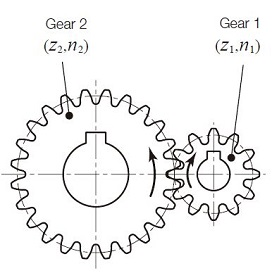(B) Bevel Gears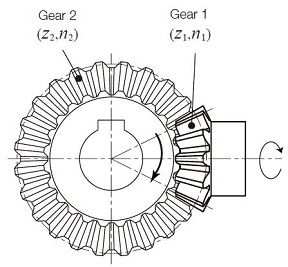(C) Spur Gear and Internal Gear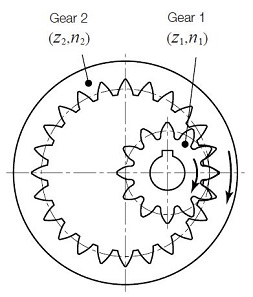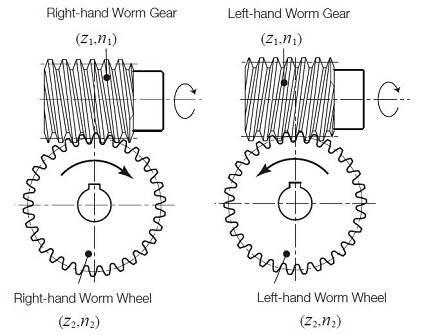In addition to these four basic forms, the combination of a rack and pinion can be considered as a specific type. The displacement of a rack, for rotation θ of the mating pinion is :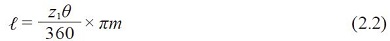Where:
πm is the reference pitch
z1 is the number of teeth of the pinion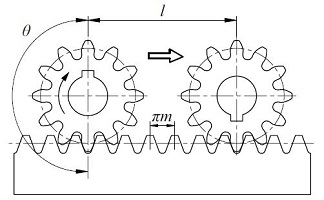Fig. 2.2 Rack and Pinionw

## 2.2 Two-Stage Gear Train

A two-stage gear train uses two single-stages in series. Figure 2.3 represents the basic form of an external gear two-stage gear train. Let the first gear in the first stage be the driver. Then the speed ratio of the two-stage gear train is :

Speed Ratio i = z2 / z1 X Z4 / Z3 = n1 / n2 x n3 / n4 (2.3)
In this arrangement, n2 = n3

In the two-stage gear train, Fig. 2.3, Gear 1 rotates in the same direction as gear 4.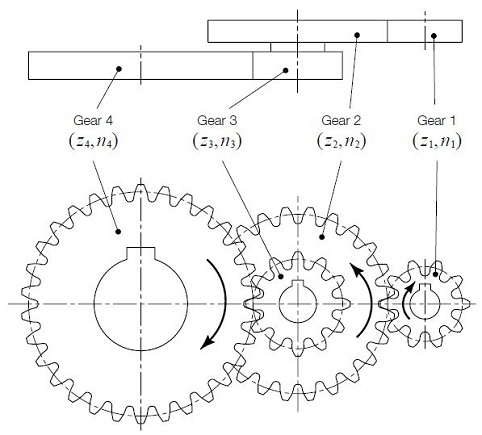Fig. 2.3 Two-Stage Gear Train

If gears 2 and 3 have the same number of teeth, then the train is simplified as shown in Figure 2.4. In this arrangement, gear 2 is known as an idler, which has no effect on the speed ratio. The speed ratio is then :

Speed ratio i = z2 / z1 x z3 / z2 = z3 / z1 (2.4)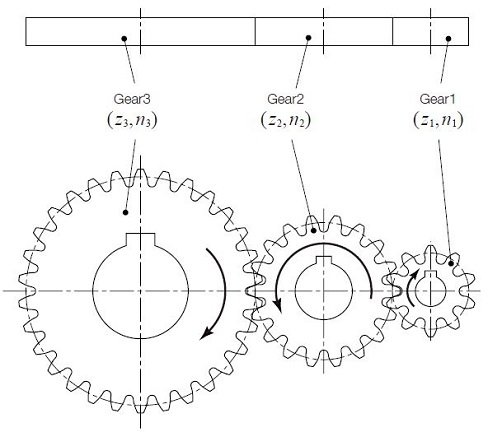Fig. 2.4 Single-stage gear train with an idler

Table 2.1 introduces calculation examples for two-stage gear trains in Fig.2.3.

Table 2.1 Speed Ratio of Two-Stage Gear Trains

 No. Term Symbols Formula Calculation Example Pinion Gear 1 Number of Teeth(First Gear) z1, z2 Set Value 10 24 2 Number of Teeth(Second Gear) z3, z4 12 30 3 RPM (Gear 1) n1 1200 – 4 Speed ratio(First Stage) i1 z2 / z1 2.4 5 Speed ratio(Second Stage) i2 z4 / z3 2.5 6 Final Speed Ratio i i1 x i2 6 7 RPM(Gear 2 and 3) n2 n1 /i1 500 8 RPM (Gear 4) n4 n1 / i – 200

RPM: Revolution per Minute
Set value here stands for the values pre-designated by the designer